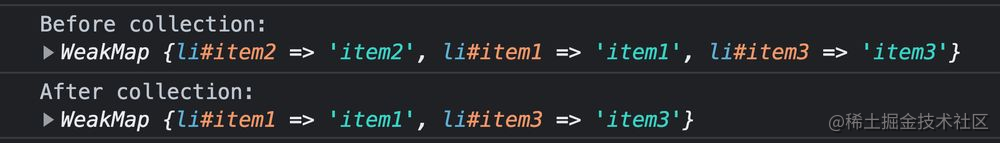# 如何使用Map处理Dom节点

``````const person = {
firstName: 'Alex',
lastName: 'MacArthur',
isACommunist: false
};
``````

``````import { ref, watchEffect } from 'vue';

let rowStates = {};
let activeRow;

document.querySelectorAll('tr').forEach((row) => {
// Set row state.
rowStates[row.id] = ref(false);

// Update row state.
if (activeRow) rowStates[activeRow].value = false;

activeRow = row.id;

rowStates[row.id].value = true;
});

watchEffect(() => {
if (rowStates[row.id].value) {
} else {
row.classList.remove('active');
}
});
});
``````

## 对象即key

``````import { ref, watchEffect } from 'vue';

- let rowStates = {};
+ let rowStates = new Map();
let activeRow;

document.querySelectorAll('tr').forEach((row) => {
-   rowStates[row.id] = ref(false);
+   rowStates.set(row, ref(false));

-       if (activeRow) rowStates[activeRow].value = false;
+       if (activeRow) rowStates.get(activeRow).value = false;

activeRow = row;

-       rowStates[row.id].value = true;
+       rowStates.get(activeRow).value = true;
});

watchEffect(() => {
-       if (rowStates[row.id].value) {
+       if (rowStates.get(row).value) {
} else {
row.classList.remove('active');
}
});
});
``````

## 读写性能更佳

`Map`必须使用哈希表或其他机制来实现，平均来说，这些机制提供的访问时间是集合中元素数量的亚线性。

“亚线性”只是意味着性能不会以与`Map`大小成比例的速度下降。因此，即使是大的Map也应该保持相当快的速度。

``````const table = document.createElement('table');
document.body.append(table);

const count = 10_000;
for (let i = 0; i < count; i++) {
const item = document.createElement('tr');
item.id = i;
item.textContent = 'item';
table.append(item);
}
``````

``````const rows = document.querySelectorAll('tr');
const times = [];
const testMap = new Map();
const testObj = {};

for (let i = 0; i < 1000; i++) {
const start = performance.now();

rows.forEach((row, index) => {
// Test Case #1
// testObj[row.id] = index;
// const result = testObj[row.id];

// Test Case #2
// testMap.set(row, index);
// const result = testMap.get(row);
});

times.push(performance.now() - start);
}

const average = times.reduce((acc, i) => acc + i, 0) / times.length;

console.log(average);
``````

100行 10000行 100000行
Object 0.023ms 3.45ms 89.9ms
Map 0.019ms 2.1ms 48.7ms
17% 39% 46%

## WeakMaps更有效地管理内存

``````<ul>
<li id="item1">first</li>
<li id="item2">second</li>
<li id="item3">third</li>
</ul>
``````

``````(async () => {
const listMap = new WeakMap();

// Stick each item in a WeakMap.
document.querySelectorAll('li').forEach((node) => {
listMap.set(node, node.id);
});

const registry = new FinalizationRegistry((heldValue) => {
// Garbage collection has happened!
console.log('After collection:', heldValue);
});

registry.register(document.getElementById('item2'), listMap);

console.log('Before collection:', listMap);

// Remove node, freeing up reference!
document.getElementById('item2').remove();

// Periodically create a bunch o' objects to trigger collection.
const obJS = [];
while (true) {
for (let i = 0; i < 100; i++) {
objs.push(...new Array(100));
}

await new Promise((resolve) => setTimeout(resolve, 10));
}
})();
``````